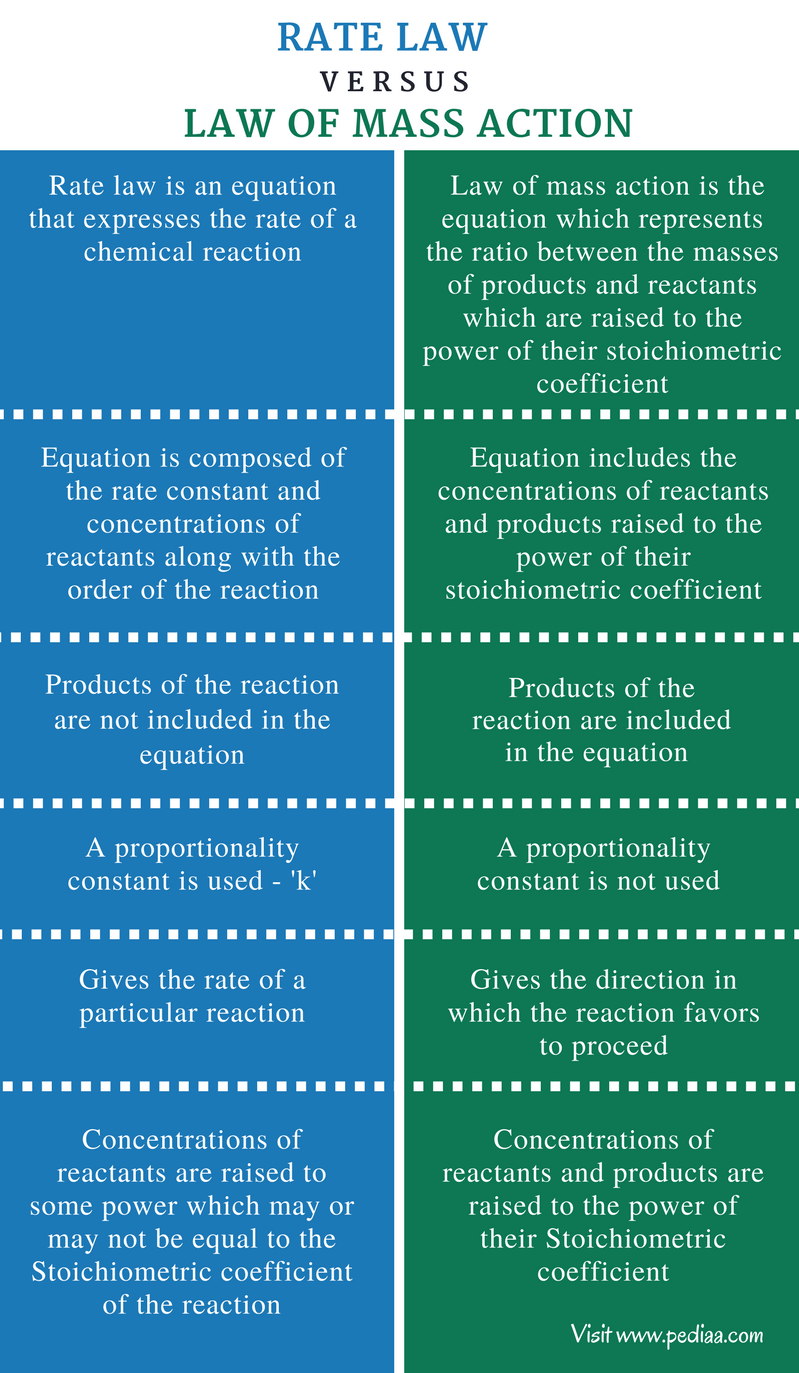# Difference Between Rate Law and Law of Mass Action

## Main Difference – Rate Law vs Law of Mass Action

Reactants and products are essential components of chemical reactions. Reactants are the chemical species that act as starting material of a chemical reaction. Reactants are often unstable and undergo different chemical reactions to become stable. Sometimes, reactants are stable, but can undergo chemical reactions when the temperature or any other parameter is changed. Products are the result of a chemical reaction. The rate law and law of mass action are two terms that describe the behavior of components in a chemical reaction. The main difference between rate law and law of mass action is that rate law is applied considering only the reactants of a reaction whereas law of mass action is applied considering both reactants and products of a reaction.

### Key Areas Covered

1. What is Rate Law
– Definition, Equation, Properties, Examples
2. What is Law of Mass Action
– Definition, Properties, Examples
3. What is the Difference Between Rate Law and Law of Mass Action
– Comparison of Key Differences

Key Terms: Law of Mass Action, Products, Rate Law, Reactants, Stoichiometric Coefficient## What is Rate Law

The rate law is an equation that expresses the rate of a chemical reaction. The components of the rate law are rate constant, reactant concentration and the order of the reaction regarding the reactant concentrations. As an example, consider that the reaction between A and B will give C as the product.

A     +      B        →       C

Therefore, the rate law of this reaction can be given as,

R    =    k[A]m[B]n

where,

R is the Rate of the reaction,

K is the rate constat,

[A] is the concentration of A,

[B] is the concentration of B,

m is the order of the reaction with respect to [A],

n is the order of the reaction with respect to [B].

The rate law indicates whether a reaction is in zero order kinetics, first order kinetic or second order kinetics. The rate constant depends only on the temperature of the system. However, the rate law does not include the concentrations or any other details about products.Figure 1: Iodometry can be used to determine the rate law of reaction between Iodine and Sodium Thiosulphate

In the case of gases, we can use the partial pressure instead of the concentration because concentration is not given for gases.

## What is Law of Mass Action

The law of mass action is the equation which represents the ratio between the masses of products and reactants which are raised to the power of their stoichiometric coefficient. However, this theory has been modified due to modern discoveries and the modification of this law represent the ratio between the concentrations of reactants and products raised to the power of their stoichiometric coefficients.

The law of mass action can be used at any circumstance. For the systems that are in equilibrium, the law of mass action is given as a constant ‘Keq” at constant conditions (such as constant temperature and pressure).

aA     +     bB          →       cC

For the above equation, the Law of mass action can be written as below, considering the concentrations of reactants and products.

Keq   =    [C]c  / [A]a[B]b

where,

Keq is the equilibrium constant,

[A] is the concentration of A and “a” is the stoichiometric coefficient of A

[B] is the concentration of B and “b” is the stoichiometric coefficient of B

[C] is the concentration of C and “c” is the stoichiometric coefficient of C

## Difference Between Rate Law and Law of Mass Action

### Definition

Rate Law: Rate law is an equation that expresses the rate of a chemical reaction.

Law of Mass Action: Law of mass action is the equation which represents the ratio between the masses of products and reactants which are raised to the power of their stoichiometric coefficient.

### Components of the Equation

Rate Law: The equation for rate law is composed of the rate constant and concentrations of reactants along with the order of the reaction.

Law of Mass Action: The equation for law of mass action includes the concentrations of reactants and products raised to the power of their stoichiometric coefficient.

### Products

Rate Law: The products of the reaction are not included in the equation for rate law.

Law of Mass Action: The products of the reaction are included in the equation for law of mass action.

### Proportionality Constant

Rate Law: A proportionality constant is used in rate law. That is the rate constant ‘k’.

Law of Mass Action: A proportionality constant is not used in law of mass action.

### Details Given

Rate Law: The rate law gives the rate of a particular reaction.

Law of Mass Action: The law of mass action gives the direction in which the reaction favors to proceed.

### Stoichiometric Coefficient

Rate Law: In rate law, the concentrations of reactants are raised to some power which may or may not be equal to the Stoichiometric coefficient of the reaction.

Law of Mass Action: In the law of mass action, the concentrations of reactants and products are raised to the power of their Stoichiometric coefficient.

### Conclusion

The rate law and the law of mass action explains the chemical kinetics of reactions. Therefore, it is very important to know the exact meaning of these terms. The main difference between rate law and law of mass action is that, rate law is given considering only the reactants of a reaction whereas law of mass action is given considering both reactants and products of a reaction.

##### References:

1.”The Rate Law – Boundless Open Textbook.” Boundless. Boundless, 26 May 2016. Web. Available here.  14 July 2017.
2.”Law of mass action.” Encyclopædia Britannica. Encyclopædia Britannica, inc., n.d. Web. Available here. 14 July 2017.

##### Image Courtesy:

1.”Iodometric titration mixture” 由LHcheM – 自己的作品 (CC BY-SA 3.0) via Commons Wikimedia